# Sound waves

Sound starts with vibrations in the air, like those produced by guitar strings, vocal cords, or speaker cones. These vibrations push nearby air molecules together, raising the air pressure slightly. The air molecules under pressure then push on the air molecules surrounding them, which push on the next set of molecules, and so on. As high-pressure areas move through the air, they leave low-pressure areas behind them. When these waves of pressure changes reach us, they vibrate the receptors in our ears, and we hear the vibrations as sound.

When you see a visual waveform that represents audio, it reflects these waves of air pressure. The zero line in the waveform is the pressure of air at rest. When the line swings up to a peak, it represents higher pressure; when the line swings down to a trough, it represents lower pressure.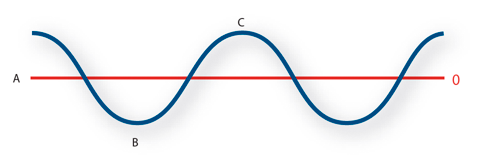A sound wave represented as a visual waveform

A. Zero line B. Low-pressure area C. High-pressure area

# Waveform measurements

Several measurements describe waveforms:

Amplitude

Reflects the change in pressure from the peak of the waveform to the trough. High-amplitude waveforms are loud; low-amplitude waveforms are quiet.

Cycle

Describes a single, repeated sequence of pressure changes, from zero pressure, to high pressure, to low pressure, and back to zero.

Frequency

Measured in hertz (Hz), describes the number of cycles per second. (For example, a 1000-Hz waveform has 1000 cycles per second.) The higher the frequency, the higher the musical pitch.

Phase

Measured in 360 degrees, indicates the position of a waveform in a cycle. Zero degrees is the start point, followed by 90º at high pressure, 180º at the halfway point, 270º at low pressure, and 360º at the end point.

Wavelength

Measured in units such as inches or centimeters, is the distance between two points with the same degree of phase. As frequency increases, wavelength decreases.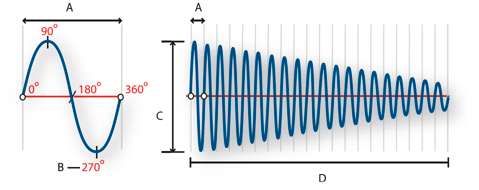A single cycle at left; a complete, 20-Hz waveform at right

A. Wavelength B. Degree of phase C. Amplitude D. One second

# How sound waves interact

When two or more sound waves meet, they add to and subtract from each other. If their peaks and troughs are perfectly in phase, they reinforce each other, resulting in a waveform that has higher amplitude than either individual waveform.

If the peaks and troughs of two waveforms are perfectly out of phase, they cancel each other out, resulting in no waveform at all.

In most cases, however, waves are out of phase in varying amounts, resulting in a combined waveform that is more complex than individual waveforms. A complex waveform that represents music, voice, noise, and other sounds, for example, combines the waveforms from each sound.

Bemærk:

Because of its unique physical structure, a single instrument can create extremely complex waves. That’s why a violin and a trumpet sound different even when playing the same note.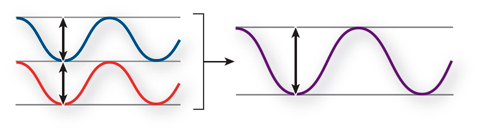In‑phase waves reinforce each other.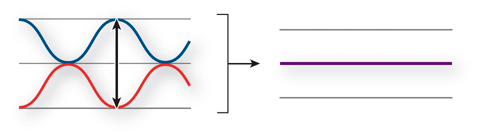Out‑of‑phase waves cancel each other out.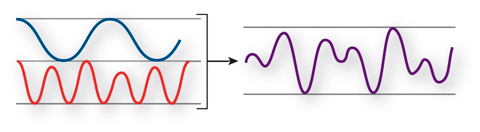Two simple waves combine to create a complex wave.Log ind på din konto